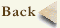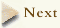State space visualization

In order to better visualize the relations between these equilibria and periodic orbits, and their relation to a typical turbulent trajectory, we have developed a new state-space visualization of moderately turbulent flows. The idea is simple, but the key ingredients -exact solutions, their stability eigenvalues and eigenvectors- were not available before.

Think of a 3D state of the fluid at a given instant -the 3D velocity field at every point of the cell- as a point in a state space. In principle this space is infinite-dimensional; in practice it is always approximated by a finite-dimensional computer mesh. This dimension is bounded from below by computational accuracy needs and the cleverness of numerical algorithms. Solutions presented here are accurate to single precision accuracy, and for this 61,506 dimensions turn out to be sufficient.

As our visual cortex can pick out and recognize large structures in these flows, not all of these 61,506 dimensions can be equally important. Can we construct a state space coordinate system in which a few coordinates captures most of these recognizable structures?

Question: “I see, this you do by POD?”